Try NerdPal! Our new app on iOS and Android

# Solve the product power $\sqrt{24\cdot 6}$

## Related Videos

Go!
Go!
1
2
3
4
5
6
7
8
9
0
a
b
c
d
f
g
m
n
u
v
w
x
y
z
.
(◻)
+
-
×
◻/◻
/
÷
2

e
π
ln
log
log
lim
d/dx
Dx
|◻|
θ
=
>
<
>=
<=
sin
cos
tan
cot
sec
csc

asin
acos
atan
acot
asec
acsc

sinh
cosh
tanh
coth
sech
csch

asinh
acosh
atanh
acoth
asech
acsch

### Videos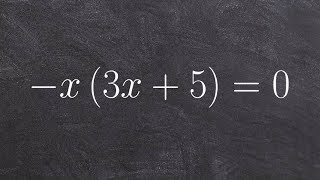### Algebra 2 - Solving a factored quadratic equation using the zero product property, -x(3x + 5) = 0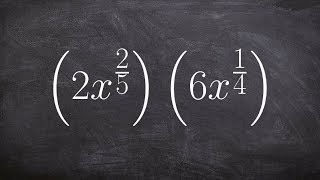### Algebra 2 - Multiplying two exponents with rational powers, then simplifying, 2x^(2/5) . 6x^(1/4)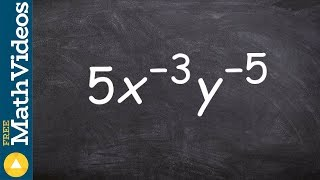### Tutorial - Rewriting exponents with positive powers to simplify an expression ex 19, 5x^-3 y^-5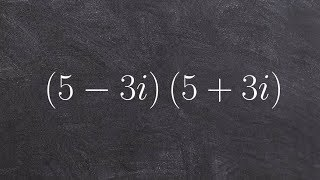### Algebra 2 - How to multiply two complex numbers using special products, (5 - 3i)(5 + 3i)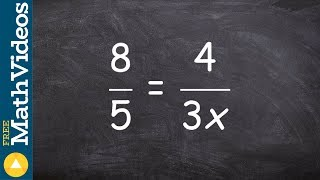### Solving a proportion using the cross product ex 7, 8/5 = (4/3x)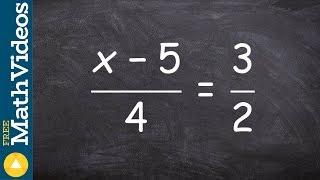SnapXam A2

### beta Got another answer? Verify it!

Go!
1
2
3
4
5
6
7
8
9
0
a
b
c
d
f
g
m
n
u
v
w
x
y
z
.
(◻)
+
-
×
◻/◻
/
÷
2

e
π
ln
log
log
lim
d/dx
Dx
|◻|
θ
=
>
<
>=
<=
sin
cos
tan
cot
sec
csc

asin
acos
atan
acot
asec
acsc

sinh
cosh
tanh
coth
sech
csch

asinh
acosh
atanh
acoth
asech
acsch

$\sqrt{24\cdot6}$